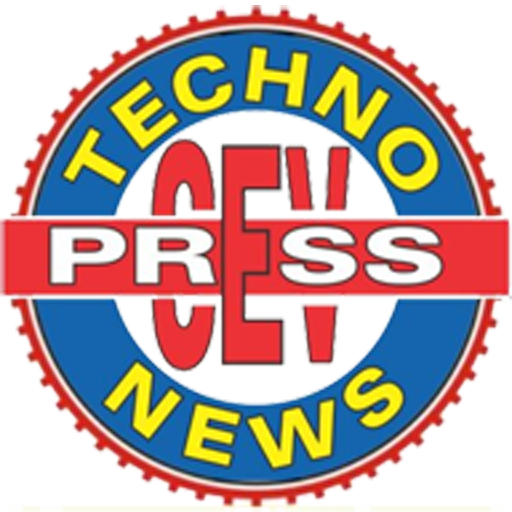# DIFFERENCE BETWEEN MARGINAL COSTING AND ABSORPTION COSTING

DIFFERENCE BETWEEN MARGINAL COSTING AND ABSORPTION COSTING

There are two alternative approaches for the valuation of inventory; they are Marginal Costing and Absorption Costing. In marginal costing, marginal cost is determined by bifurcating fixed cost and variable cost. Only variable costs are charged to operation, whereas the fixed cost is excluded from it and are charged to profit and loss account for the period.

Marginal Costing

A marginal cost is the cost of one additional unit of output. Marginal costing is a costing technique whereby the marginal cost is charged to units of costs while the fixed cost is completely written off against the contribution.

Marginal costing is helpful in certain decision-making in a business on matters such as whether to carry on with a service or product, replacement of machinery, and in ascertaining the appropriate level of activity, through the break-even analysis. This helps in the reflection on how the overall profit is affected by the decrease or increase in production levels.

In marginal costing;

• The prices are determined on the basis of marginal contribution and marginal cost
• Costs involved are variable and fixed costs and are classified on the basis variability
• The profitability of a product is based on the contribution margin
• Only variable costs are taken into account when valuing the finished goods and work in progress

• Fixed costs are classified as a period cost and are charged in full to the period in mention
• It is helpful in the decision-making process
• It prevents under or over-absorption of overheads
• Contribution per unit is constant and does not change in change volumes
• It is simple to operate

Absorption Costing

Also referred to as full costing, it is a costing system whereby all manufacturing costs, including variable and fixed costs, are assumed to be product costs. The period costs, in this case, include administrative, selling and general costs which do not go into the cost of the product but are expensed at the period incurred. The product costs including variable manufacturing overhead, direct labor, fixed manufacturing overhead, and direct material are costs that go into the product.

The advantages associated with absorption costing include;

• It is GAAP(Generally Accepted Accounting Principles) compliant
• Takes into account all production costs
• It helps in the estimation of job costs and profits on jobs by absorbing overheads into the costs of products.

## Differences Between Marginal Costing and Absorption Costing

1. The costing method in which variable cost is apportioned exclusively, to the products is known as Marginal Costing. Absorption Costing is a costing system in which all the costs are absorbed and apportioned to products.
2. In Marginal Costing, Product related costs will include only variable costs while in the case of Absorption costing, fixed cost is also included in product-related cost apart from variable cost.
3. Marginal Costing divides overheads into two broad categories, i.e. Fixed Overhead and Variable Overhead. Look at the other term Absorption costing, which classifies overheads into the following three categories Production, Administration, and Selling & Distribution.
4. In marginal costing profit can be ascertained through the help of Profit Volume Ratio [(Contribution / Sales) * 100]. On the other hand, Net Profit shows the profit in the case of Absorption Costing.
5. In Marginal Costing variances in the opening and closing stock will not influence the per-unit cost. Unlike Absorption Costing, where the variances between the stock at the beginning and the end will show their effect by increasing/decreasing per-unit cost.
6. In marginal costing, the cost data is presented to outline the total cost of each product. On the contrary, in absorption costing, the cost data is presented in the traditional way, the net profit of each product is ascertained after deducting fixed costs along with their variable cost.
error: Content is protected !!